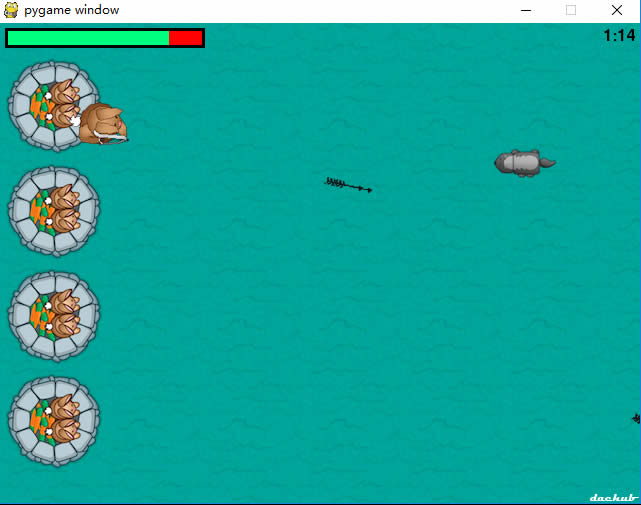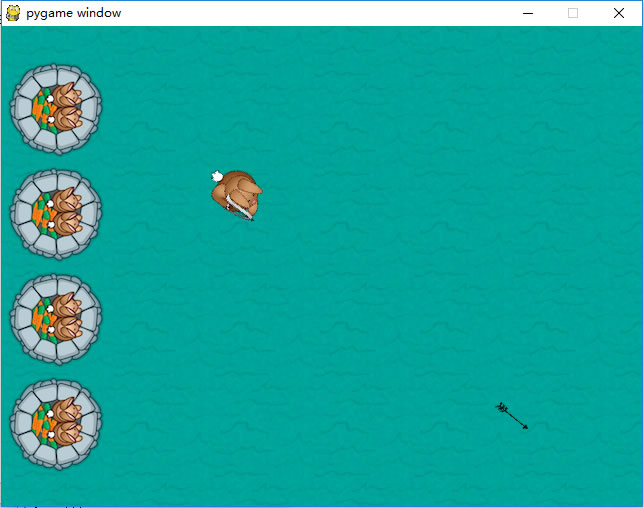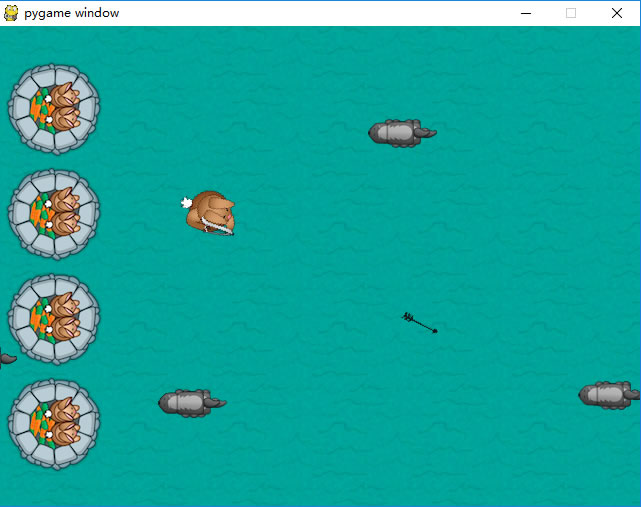```acc=[0,0]
arrows=[]```

`arrow = pygame.image.load (“resources/images/bullet.png”)`

```position=pygame.mouse.get_pos()
acc+=1
arrows.append([math.atan2(position-(playerpos1+32),position-(playerpos1+26)),playerpos1+32,playerpos1+32])```

```# 6.2 - Draw arrows
for bullet in arrows:
index=0
velx=math.cos(bullet)*10
vely=math.sin(bullet)*10
bullet+=velx
bullet+=vely
if bullet<-64 or bullet>640 or bullet<-64 or bullet>480:
arrows.pop(index)
index+=1
for projectile in arrows:
arrow1 = pygame.transform.rotate(arrow, 360-projectile*57.29)
screen.blit(arrow1, (projectile, projectile))```###### 图4

```badtimer=100
healthvalue=194```

```badguyimg1 = pygame.image.load("resources/images/badguy.png")

```# 6.3 – Draw moles
else:
index=0
index+=1

`import random`

`badtimer-=1`###### 图5

```# 6.3.1 - Attack castle
healthvalue -= random.randint(5,20)

```        #6.3.2 - Check for collisions
index1=0
for bullet in arrows:
bullrect=pygame.Rect(arrow.get_rect())
bullrect.left=bullet
bullrect.top=bullet
acc+=1
arrows.pop(index1)```

```    # 6.4 - Draw clock
font = pygame.font.Font(None, 24)
survivedtext = font.render(str((90000-pygame.time.get_ticks())/60000)+":"+str((90000-pygame.time.get_ticks())/1000%60).zfill(2), True, (0,0,0))
textRect = survivedtext.get_rect()
textRect.topright=[635,5]
screen.blit(survivedtext, textRect)```

```healthbar = pygame.image.load("resources/images/healthbar.png")

```# 6.5 - Draw health bar
screen.blit(healthbar, (5,5))
for health1 in range(healthvalue):
screen.blit(health, (health1+8,8))```

```    #10 - Win/Lose check
if pygame.time.get_ticks()>=90000:
running=0
exitcode=1
if healthvalue<=0:
running=0
exitcode=0
if acc!=0:
accuracy=acc*1.0/acc*100
else:
accuracy=0
# 11 - Win/lose display
if exitcode==0:
pygame.font.init()
font = pygame.font.Font(None, 24)
text = font.render("Accuracy: "+str(accuracy)+"%", True, (255,0,0))
textRect = text.get_rect()
textRect.centerx = screen.get_rect().centerx
textRect.centery = screen.get_rect().centery+24
screen.blit(gameover, (0,0))
screen.blit(text, textRect)
else:
pygame.font.init()
font = pygame.font.Font(None, 24)
text = font.render("Accuracy: "+str(accuracy)+"%", True, (0,255,0),)
textRect = text.get_rect()
textRect.centerx = screen.get_rect().centerx
textRect.centery = screen.get_rect().centery+24
screen.blit(youwin, (0,0))
screen.blit(text, textRect)
while 1:
for event in pygame.event.get():
if event.type == pygame.QUIT:
pygame.quit()
exit(0)
pygame.display.flip()```

```gameover = pygame.image.load("resources/images/gameover.png")

```# 4 – keep looping through
running = 1
exitcode = 0
while running:```

pygame让添加音效变得十分简单，首先，需要在#2程序段初始化音效模块mixer：

`pygame.mixer.init()`

```# 3.1 - Load audio
hit = pygame.mixer.Sound("resources/audio/explode.wav")
enemy = pygame.mixer.Sound("resources/audio/enemy.wav")
shoot = pygame.mixer.Sound("resources/audio/shoot.wav")
hit.set_volume(0.05)
enemy.set_volume(0.05)
shoot.set_volume(0.05)
pygame.mixer.music.play(-1, 0.0)
pygame.mixer.music.set_volume(0.25)```

```# section 6.3.1 after if badrect.
left<64:
hit.play()
# section 6.3.2 after if badrect.
colliderect(bullrect):
enemy.play()
# section 8, after if event.
type==pygame.MOUSEBUTTONDOWN:
shoot.play()```